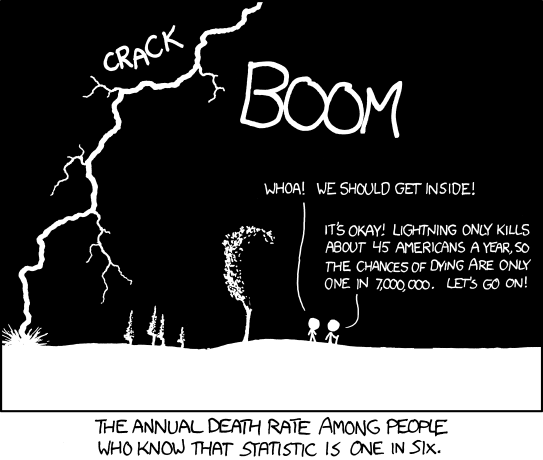# Probably not measurement.

• Posted on: 11 October 2016
• By: admin
Block:
Term:
Year:
LA Code:
Code:
Room:
Learning Area:
Levels:
3
4

So in this class we will probably learn some measurement and some probability.Learn about calculating simple probabilities. We might have to revise fractions also.

Investigate simple situations that involve elements of chance by comparing experimental results with expectations from models of all the outcomes, acknowledging that samples vary.

Use simple fractions and percentages to describe probabilities.

Understand the metric system and use estimation to answer important questions like how many balloons would it take to fill the School Office?